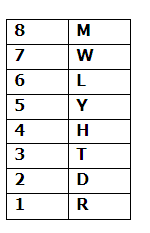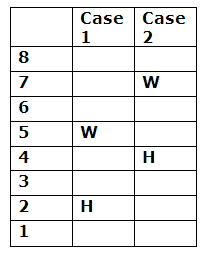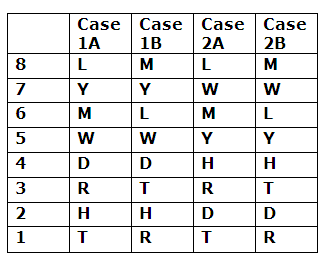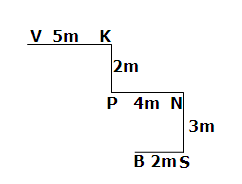# IBPS RRB Clerk/EPFO Assistant/LIC ADO Prelims Reasoning Ability Questions 2019 (Day-5)

Dear Aspirants, Our IBPS Guide team is providing new series of Reasoning Questions for IBPS RRB Clerk/EPFO Assistant/LIC ADO Prelims Reasoning Ability Questions 2019 (Day-03) Prelims 2019 so the aspirants can practice it on a daily basis. These questions are framed by our skilled experts after understanding your needs thoroughly. Aspirants can practice these new series questions daily to familiarize with the exact exam pattern and make your preparation effective.

Check here for IBPS RRB Clerk Prelims Mock Test 2019

Check here for IBPS EPFO Assistant Prelims Mock Test 2019

Check here for LIC ADO Prelims Mock Test 2019

[WpProQuiz 6627]

Direction (1-5): Study the following information carefully and answer the questions given below:

Eight persons L, R, T, M, H, W, D and Y are living in eight different floors of a building. The lowermost floor is numbered 1, the one above floor is numbered 2, and so on till the topmost floor is numbered 8 but not necessarily in the same order. Persons name starts with first half of the English alphabetical series lives on even numbered floors and the remaining person lives on odd numbered floors.

Two persons live between H and W. L lives above T. Two persons live between Y and D. D lives below Y. W lives above H. Number of persons live above L is same as the number of persons live below T. M does not live immediately above or immediately below Y. R lives below M.

1) Which of the following person lives immediately above Y?

a) M

b) H

c) L

d) W

e) None of these

2) How many persons live between Y and R?

a) None

b) One

c) Two

d) Three

e) None of these

3) Which of the following person lives on lowermost floor?

a) M

b) W

c) T

d) R

e) None of these

4) Four of the following five are alike in a certain way and hence they form a group. Which one of the following does not belong to that group?

a) M-Y

b) L-T

c) D-Y

d) H-W

e) R-L

5) If M is related to R, T is related to L, in the same way D is related to which of the following?

a) H

b) Y

c) W

d) L

e) None of these

Direction (6-8): In these questions, relationship between different elements is shown in the statements. These statements are followed by two conclusions.

a) If only conclusion I follows

b) If only conclusion II follows.

c) If either conclusion I or II follows.

d) If neither conclusion I nor II follows.

e) If both conclusion I and II follow.

6) Statements: T ≥ K > P ≥ Y ≥ W > S > H

Conclusions:

I) W < T

II) S ≤ Y

7) Statements: H ≤ P ; P > L ; L = O ; O ≥ N

Conclusions:

I) P < N

II) P = N

8) Statements: K = T ; T ≤ P ≤ W ; F > K

Conclusions:

I) F < P

II) W ≥ K

Direction (9-10): Study the following information carefully and answer the questions given below:

Point V is 5m to the west of Point K. Point P is 2m to the south of Point K. Point N is 4m to the east of Point P. Point S is 3m to the south of Point N. Point B is 2m to the west of Point S.

9) What is the shortest distance between Point S and Point P?

a) 4m

b) 3m

c) 5m

d) 6m

e) None of these

10) Point B is in which direction with respect to Point V?

a) South West

b) North West

c) North East

d) South East

e) None of these

Direction (1-5):D, H, L and M live on even numbered floor whereas R, T, W and Y live on odd numbered floor.

Two persons live between H and W. W lives above H. H can live either second or fourth floor.Number of person lives above L is same as the number of person lives below T. L lives above T. Two persons live between Y and D. D lives below Y. M do not live immediately above or below Y. R lives below M.Case 1A, 1B and 2A will be dropped because M do not live immediately above or below Y.Direction (6-8) :

I) T ≥ K > P ≥ Y ≥ W -> True

II) Y ≥ W > S -> False

I) P > L = O ≥ N  -> False

II) P > L = O ≥ N -> False

I) F > K = T ≤ P -> False

II) K = T ≤ P ≤ W -> True

Direction (9-10):